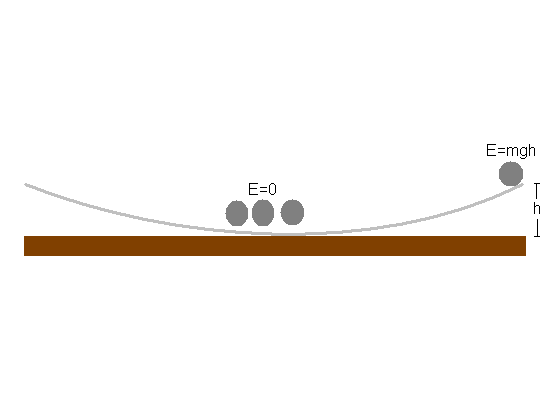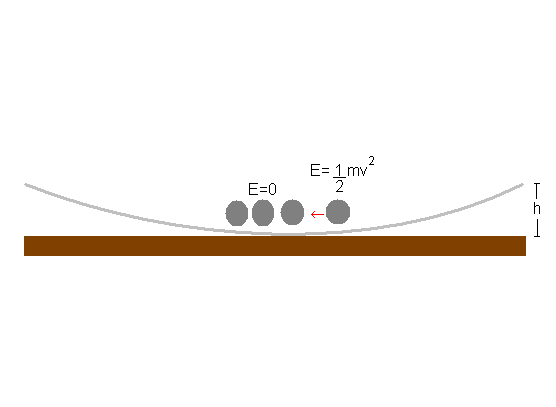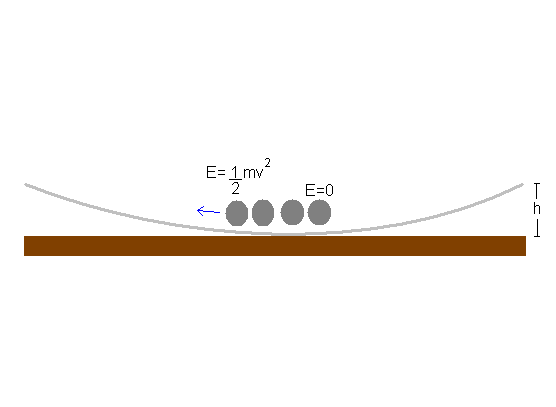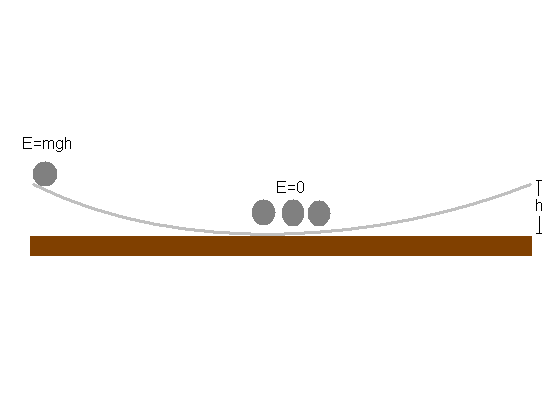### When a magnetic ball rolls down the same ramp, it launches the other ball completely off the ramp!

Watch Video:

Teachable Topics:

• Conservation of Energy
• Conservation of Momentum
• Magnetism

Theory:

Two of the fundamental principals in physics and in life are that energy and momentum are conserved. In this experiment, those principles are demonstrated, as energy is converted from one form to another.

By splitting the motion of the ball into two parts, it becomes easier to analyze. The first part will be from when the ball is released at the top of the ramp, to immediately before the collision. The second part will be from the collision to the point where the next ball reaches its maximum potential energy (at the maximum height the ball reaches).

When the steel ball is at rest at the top of the ramp, it has zero kinetic energy, but it has potential energy that is equal to its mass multiplied by the height above the bottom of the ramp, multiplied by the acceleration due to gravity (E=mgh). The balls at the bottom of the ramp have zero kinetic energy and zero potential energy.As the ball at the top is released, its potential energy is converted into potential energy, and immediately before the collision with the other balls, it has kinetic energy of 1/2*m*v2 and zero potential energy. This final kinetic energy is equal to the initial potential energy (or if friction is taken into account, kinetic + energy lost due to friction = initial potential energy)When the collision occurs, the kinetic energy from the first ball is transferred to the ball on the end. In an ideal situation, there would be no energy loss during the collision, however, realistically, there is a small amount that is lost in the transfer as it is not a perfectly elastic collision. For this experiment, we neglect the energy lost during the collision, and assume all of the kinetic energy is transferred. As the ball on the end starts to move, its kinetic energy is converted into potential energy.As the ball reaches its maximum height, potential energy is at a maximum, and kinetic energy is zero. If energy is conserved the ball will have reached the same height the original ball was released from.For the magnetic ball, the situation is a little different. The ball's energy still changes from potential to kinetic, but as the magnetic ball rolls towards the steel ball, the magnetic force causes the ball to accelerate a small amount, so that the kinetic energy just before the impact is slightly larger than it was for the steel ball in the first situation. Since the kinetic energy right before the collision was larger, the kinetic energy right after the collision must be too. This is why the ball launches off the ramp. If the ramp had been higher, the second ball would have reached a higher point than the magnetic ball was released from.

Apparatus:
• Magnetic Accelerator Apparatus
• 4 Steel Balls
• 1 Magnetic Ball

Procedure:

1. Line up 3 steel balls in the middle of the track.
2. Release a steel ball from the top of the track, allowing it to collide with the other three.
3. Reset balls.
4. Release the magnetic ball from the top of the track and allow it to collide with the three steel balls on the track.
5. Watch as the steel ball on the end is launched off the track.